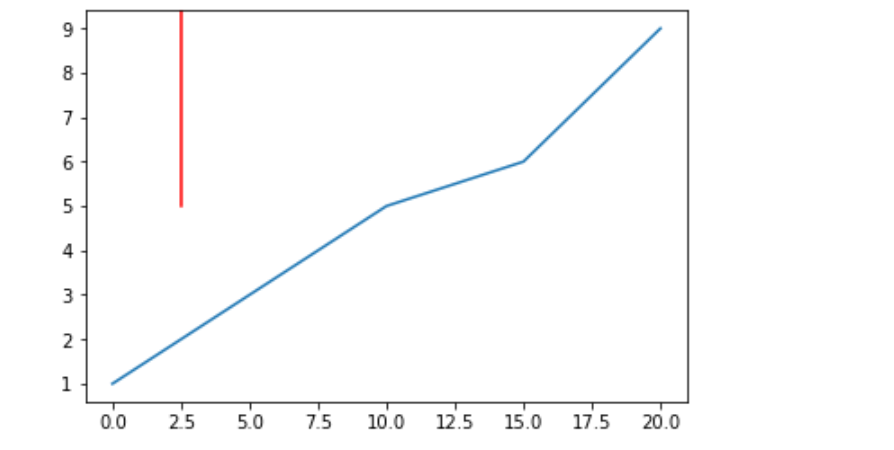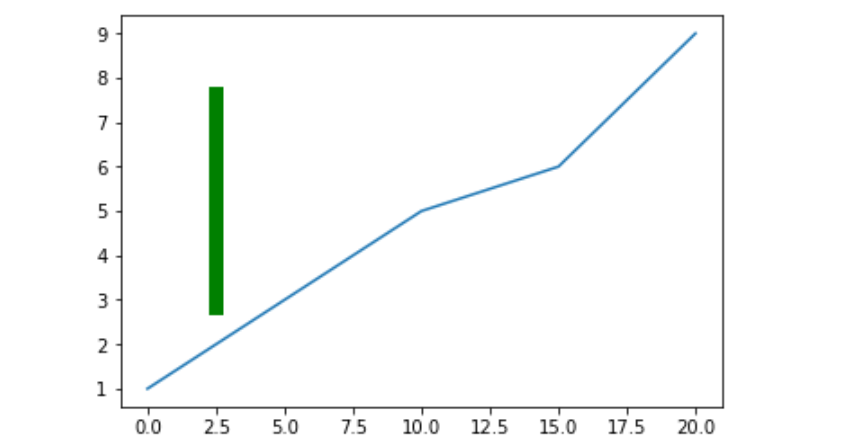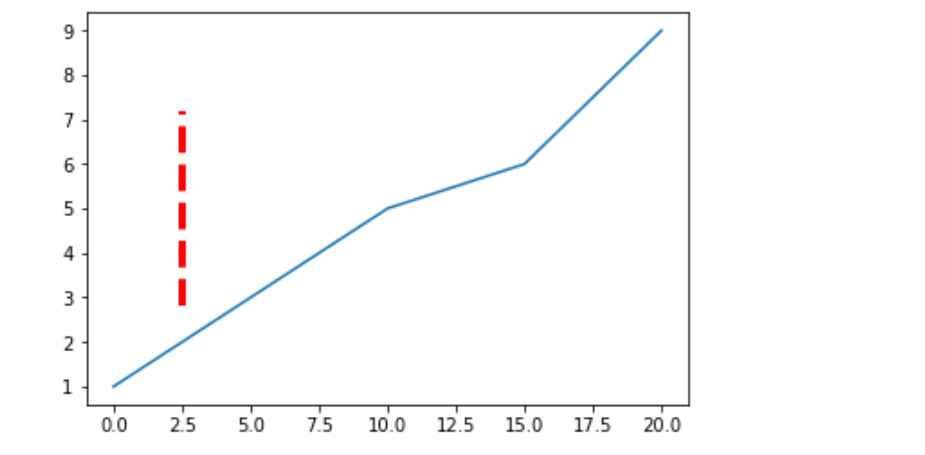Matplotlib.pyplot.axvline() in Python

• Last Updated : 21 Apr, 2020

Matplotlib is a plotting library for creating static, animated, and interactive visualizations in Python. Matplotlib can be used in Python scripts, the Python and IPython shell, web application servers, and various graphical user interface toolkits like Tkinter, awxPython, etc.

Pyplot is a Matplotlib module which provides a MATLAB-like interface. Matplotlib is designed to be as usable as MATLAB, with the ability to use Python and the advantage of being free and open-source.

matplotlib.pyplot.axvline()

This function add the vertical lines across the axes of the plot

Syntax:
matplotlib.pyplot.axvline(x=0, ymin=0, ymax=1, **kwargs)

Parameters:
x : x position in data coordinates to place the vertical line
ymin :vertical line starting position on y axis, it will take values between 0 and 1, 0 being bottom of the axis, 1 being top of the axis
ymax :vertical line ending position on y axis, it will take values between 0 and 1, 0 being bottom of the axis, 1 being top of the axis
**kwargs :Other optional parameters to change the properties of the line, like
changing color, linewidth etc

Example-1:

 # Importing matplotlib.pyplot as pltimport matplotlib.pyplot as plt    # Initialising values of x and yx =[0, 5, 10, 15, 20]y =[1, 3, 5, 6, 9]  # Plotting the graphplt.plot(x, y)  # Drawing red vertical line at# x = 2.5 starting at half the #length of y axis(ymin = 0.5) and #continuing till the end(ymax = 1)# And setting the color of line to redplt.axvline(x = 2.5, ymin = 0.5, ymax = 1,            color ='red')  plt.show()

Output :Example-2 :

 import matplotlib.pyplot as plt  x =[0, 5, 10, 15, 20]y =[1, 3, 5, 6, 9]  plt.plot(x, y)# Drawing vertical line from 25 % # of the y-axis length to 80 % # And also increasing the linewidthplt.axvline(x = 2.5, ymin = 0.25, ymax = 0.80,            linewidth = 8, color ='green')  plt.show()

Output:Example-3 :

 import matplotlib.pyplot as plt  x =[0, 5, 10, 15, 20]y =[1, 3, 5, 6, 9]  plt.plot(x, y)  # Drawing vertical line from 25 %# of the y-axis length to 75 % # And also changing the linestyleplt.axvline(x = 2.5, ymin = 0.25, ymax = 0.75,            linewidth = 4, linestyle ="--",            color ='red')  plt.show()

Output:My Personal Notes arrow_drop_up